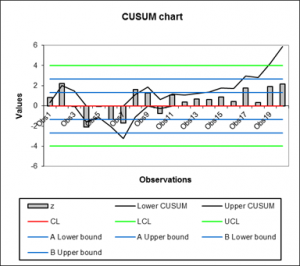# Ponderación por el tiempo

Time Weighted charts are quality control charts based on transformed - smoothed or normalized - data. Draw them in Excel using the XLSTAT software.## What are time weighted charts

Time weighted charts are a type quality control charts used in the area of good production. They are used to supervise production quality, in the case where you have a group of measurements or a single measurement for each point in time. The measurements need to be made on quantitative variables.

The weighted time charts are useful to recap the mean and the variability of the measured production quality.

## Time weighted charts in XLSTAT

XLSTAT time weighted charts tool offers you the following chart types:

• CUSUM (Cumulative sum of quality characteristic measurement) or CUSUM individual
• UWMA (Uniformly weighted moving average) or UWMA individual
• EWMA (Exponentially weighted moving average) or EWMA individual

A CUSUM, UWMA or EWMA chart is useful to follow the mean of a production process. Mean shifts are easily visible in the diagrams.

### UWMA and EWMA charts

These charts are not directly based on the raw data. They are based on the smoothed data. In the case of UWMA charts, the data is smoothed using a uniform weighting in a moving window. Then the chart is analyzed like Shewhart charts. In the case of EWMA charts, the data is smoothed using a exponentially weighting. Then the chart is analyzed like Shewhart charts.

### CUSUM charts

These charts are not directly based on the raw data. They are based on the normalized data. These charts help to detect mean shifts of a user defined granularity. The granularity is defined by the design parameter k. K is the half of the mean shift to be detected. Normally to detect 1 sigma shifts, k is set to 0.5. Two kinds of CUSUM charts can be drawn: one and two sided charts.### analice sus datos con xlstat

prueba gratuita de 14 días

Incluido en